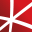# Top 20 NuGet fit Packages

Math.NET Numerics is the numerical foundation of the Math.NET project, aiming to provide methods and algorithms for numerical computations in science, engineering and every day use. Supports .NET 5.0 or higher, .NET Standard 2.0 and .NET Framework 4.6.1 or higher, on Windows, Linux and Mac. This pac...
Math.NET Numerics is the numerical foundation of the Math.NET project, aiming to provide methods and algorithms for numerical computations in science, engineering and every day use. Supports .NET 5.0 or higher, .NET Standard 2.0 and .NET Framework 4.6.1 or higher, on Windows, Linux and Mac.
F# Modules for Math.NET Numerics, the numerical foundation of the Math.NET project, aiming to provide methods and algorithms for numerical computations in science, engineering and every day use. Supports .NET 5.0 or higher, .NET Standard 2.0 and .NET Framework 4.6.1 or higher, on Windows, Linux and ...
Foundational classes for financial, engineering, and scientific applications, including complex number classes, general vector and matrix classes, structured sparse matrix classes and factorizations, general sparse matrix classes and factorizations, general matrix decompositions, least squares solut...
The GPU-accelerated version of package CenterSpace.NMath. With a few minor exceptions, such as optional GPU configuration settings, the API is identical between CenterSpace.NMath.Premium and CenterSpace.NMath. If using at least .NET Framework 4.6.1 or .NET Core 2.0, we recommend using one of our NMa...
The Extreme Optimization Numerical Libraries for .NET are a set of libraries for numerical computing and data analysis. This is the main package that contains all the core functionality. For optimal performance, we strongly recommend also referencing one of the native packages based on Intel's Mat...
F# Modules for Math.NET Numerics, the numerical foundation of the Math.NET project, aiming to provide methods and algorithms for numerical computations in science, engineering and every day use. Supports .NET 5.0 or higher, .NET Standard 2.0 and .NET Framework 4.6.1 or higher, on Windows, Linux and ...
Foundational classes for financial, engineering, and scientific applications, including complex number classes, general vector and matrix classes, structured sparse matrix classes and factorizations, general sparse matrix classes and factorizations, general matrix decompositions, least squares solut...
Class library implementing advanced mathematical algorithms, transforms, and time series manipulations. Implementations favour simplicity and correctness.
fitSharp is a set of open-source functional testing tools for .NET, inspired by the Framework for Integrated Test
Foundational classes for financial, engineering, and scientific applications, including complex number classes, general vector and matrix classes, structured sparse matrix classes and factorizations, general sparse matrix classes and factorizations, general matrix decompositions, least squares solut...
Foundational classes for financial, engineering, and scientific applications, including complex number classes, general vector and matrix classes, structured sparse matrix classes and factorizations, general sparse matrix classes and factorizations, general matrix decompositions, least squares solut...## NMath - Standard Library - Windows and Linux - x64

Foundational classes for financial, engineering, and scientific applications, including complex number classes, general vector and matrix classes, structured sparse matrix classes and factorizations, general sparse matrix classes and factorizations, general matrix decompositions, least squares solut...## NMath - Standard Library - Windows - x86 and x64

Foundational classes for financial, engineering, and scientific applications, including complex number classes, general vector and matrix classes, structured sparse matrix classes and factorizations, general sparse matrix classes and factorizations, general matrix decompositions, least squares solut...
Foundational classes for financial, engineering, and scientific applications, including complex number classes, general vector and matrix classes, structured sparse matrix classes and factorizations, general sparse matrix classes and factorizations, general matrix decompositions, least squares solut...
The Extreme Optimization Numerical Libraries for .NET are a set of libraries for numerical computing and data analysis. This package contains the single-precision mixed-mode native provider. This is the recommended native provider for the classic .NET Framework on Windows. Supports .NET Framework ...
The Extreme Optimization Numerical Libraries for .NET are a set of libraries for numerical computing and data analysis. This package contains the single-precision mixed-mode native provider. This is the recommended native provider for the classic .NET Framework on Windows. Supports .NET Framework ...
The Extreme Optimization Numerical Libraries for .NET are a set of libraries for numerical computing and data analysis. This package contains the mixed-mode native provider. This is the recommended native provider for the classic .NET Framework on Windows. Supports .NET Framework 4.0 and 4.6+ on W...
The Extreme Optimization Numerical Libraries for .NET are a set of libraries for numerical computing and data analysis. This package contains the mixed-mode native provider. This is the recommended native provider for the classic .NET Framework on Windows. Supports .NET Framework 3.5 on Windows.
Extreme Optimization Numerical Libraries for .NET P/Invoke MKL Provider for Windows.